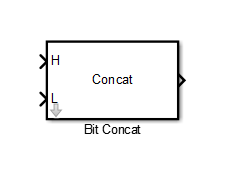# Bit Concat

Concatenates up to 128 input words into single output

•Libraries:
HDL Coder / Logic and Bit Operations

## Description

The Bit Concat block concatenates up to 128 input words into a single output. The input port labeled `L` designates the lowest-order input word. The input port labeled `H` designates the highest-order input word. For scalar inputs, two or more input signals should be connected to the block. For vector inputs, at least one input should be connected to the block. The block uses the `bitconcat` function such that the right-to-left ordering of words in the output follows the low-to-high ordering of input signals. To learn how the block operates, see Algorithms.

## Ports

### Input

expand all

The highest-order input word for the block to concatenate that becomes the left-most value in the concatenated output signal.

Data Types: `int8` | `int16` | `int32` | `int64` | `uint8` | `uint16` | `uint32` | `uint64` | `Boolean` | `fixed point`

The lowest-order input word for the block to concatenate that becomes the right-most value in the concatenated output signal.

Data Types: `int8` | `int16` | `int32` | `int64` | `uint8` | `uint16` | `uint32` | `uint64` | `Boolean` | `fixed point`

### Output

expand all

The output is the concatenated word in low-to-high ordering of the input words. The maximum concatenated output word size is 128 bits.

Data Types: `int8` | `int16` | `int32` | `int64` | `uint8` | `uint16` | `uint32` | `uint64` | `fixed point`

## Parameters

expand all

Enter an integer specifying the number of input signals. The number of block input ports updates when you change Number of Inputs.

• Default: 2

• Minimum: 1

• Maximum: 128

Caution

Make sure that the Number of Inputs is equal to the number of signals you connect to the block. If the block has unconnected inputs, an error occurs during code generation.

#### Programmatic Use

 Block parameter: `numInputs` Type: string scalar | character vector Value: A positive integer value less than or equal to 128 Default: `'2'`

## Algorithms

The block uses the `bitconcat` function to compute the result. How the block operates depends on the number and dimensions of the inputs, as follows:

• Single input: The input is a scalar or a vector. When the input is a vector, the code generator concatenates the individual vector elements. For example, if the input vector is `[1 2]` that has the data type `ufix4`, the output concatenates the elements `1` and `2` such that `1` forms the MSB (Most Significant Bit). The output is:

y = dec2bin('00010010') = 18

• Two inputs: Inputs are any combination of scalar and vector.

• When one input is scalar and the other is a vector, the code generator performs scalar expansion. Each vector element is concatenated with the scalar, and the output has the same dimension as the vector. For example, consider a vector `[1 2]` input to the `H` port and a scalar value `3` as input to the `L` port. Both inputs have the data type `ufix4`. The output is a vector that concatenates such that the MSB is a concatenation of elements `1` and `3`, and the LSB is a concatenation of elements `2` and `3`.

y = [dec2bin('00010011') dec2bin('00100100')] = [19 35]

• When both inputs are vectors, they must have the same size. In this case, the last element is the lowest-order word and the first element is the highest order word. For example, consider two input vectors `[1 2]` and `[3 4]` that have the data type `ufix4`. The output is a vector that concatenates such that the MSB is a concatenation of elements `1` and `3`, and the LSB is a concatenation of elements `2` and `4`.

y = [dec2bin('00010011') dec2bin('00100100')] = [19 36]

• Three or more inputs (up to a maximum of `128` inputs): Inputs are uniformly scalar or vector. All vector inputs must have the same size. For example, consider three vector inputs `[1 2]`, `[3 4]`, and `[5 6]` such that vector ```[1 2]``` is input to the `H` port and `[5 6]` is input to the `L` port. In this case, the output is a vector that first concatenates `[1 2]` and `[3 4]`.

temp = [dec2bin('00010011') dec2bin('00100100')] = [19 36]

The result of this computation is then concatenated with the vector ```[5 6]``` to produce the output.

y = [dec2bin('000100110101') dec2bin('001001000110')] = [309 582]

## Version History

Introduced in R2014a